# AP Physics 1 Question 309: Answer and Explanation

### Test Information

Question: 309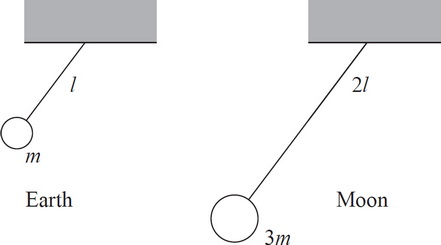7. A pendulum with a ball of mass m hanging from a string of length l is set in motion on Earth, and the system is found to have a frequency of f. If the length of the string were doubled, the hanging mass tripled, and the system moved to the moon, what would be the new frequency?

NOTE: Acceleration due to gravity of the Moon is approximately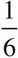of Earth's.

• A.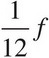• B.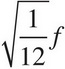• C.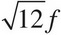• D. 12f

The frequency of a pendulum is f =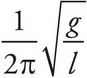. Therefore, the change in mass would have no effect on the system, and the others would change the equation to f =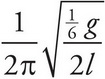. Thus, the original frequency f will become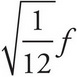.